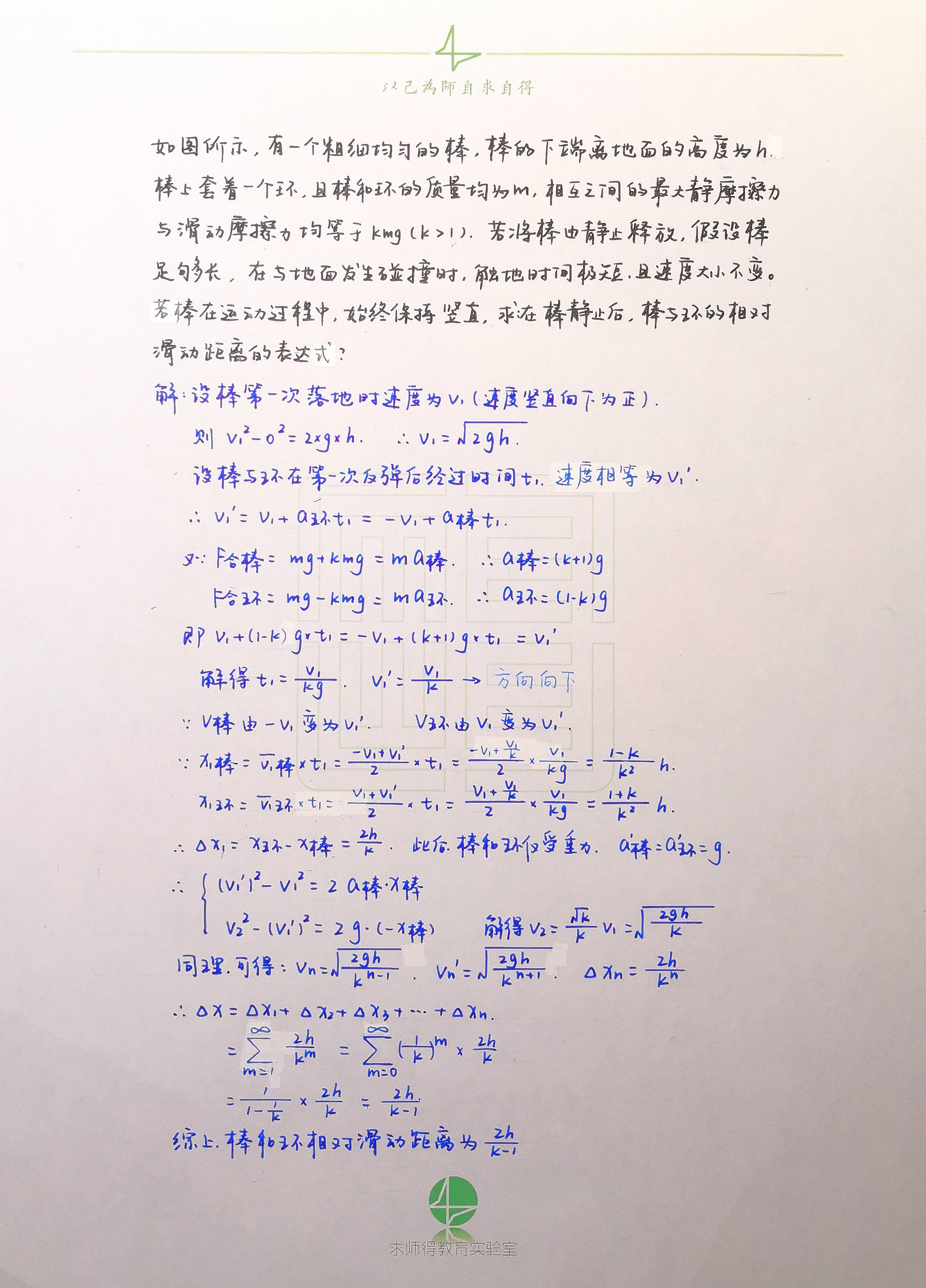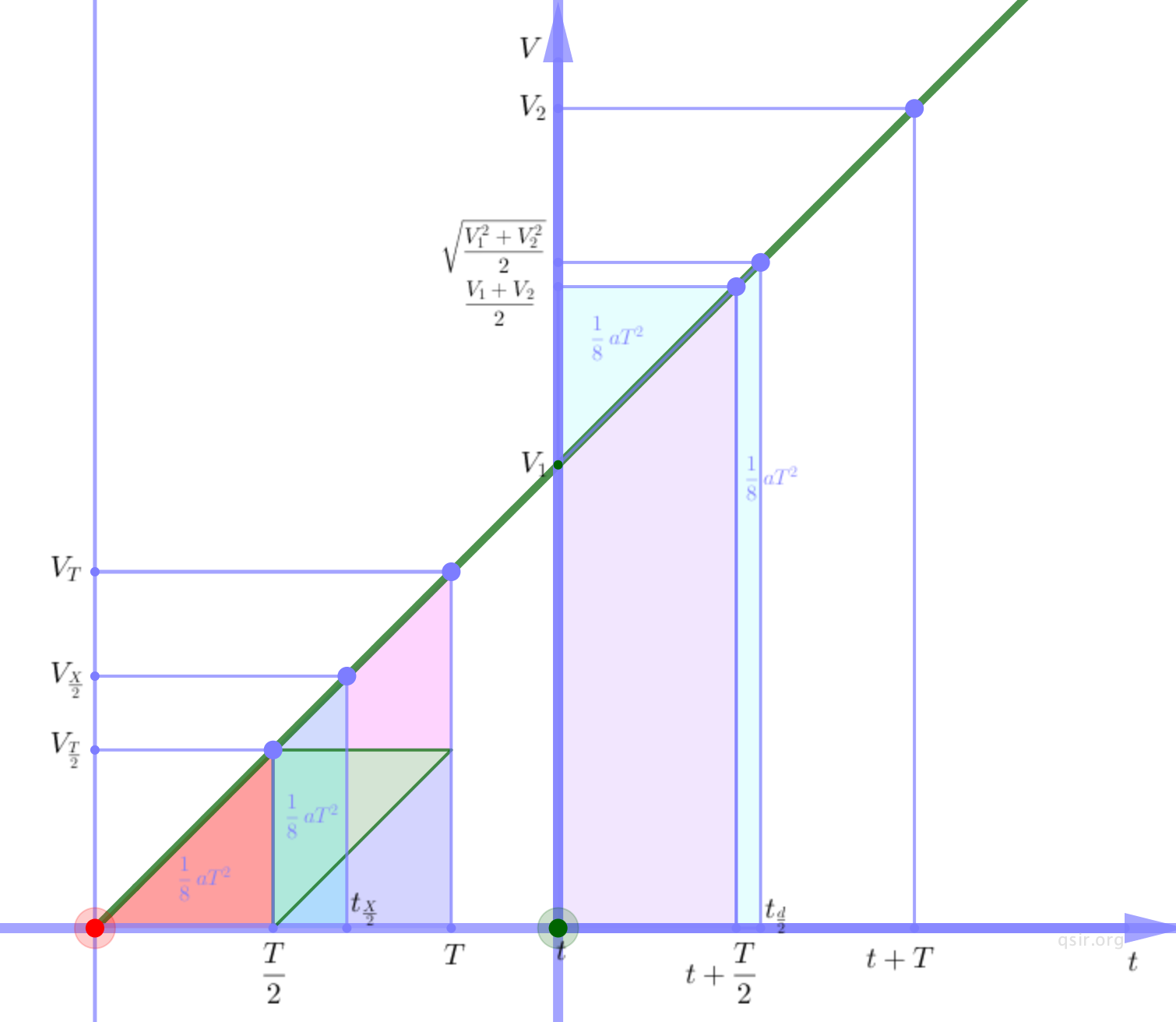29

## 位移中点与时间中点的位移$\Delta{x}=\frac{v_{\frac{x}{2}}^2-v_{\frac{t}{2}}^2}{2a}$，整理的，$\Delta{x}=\frac{(v_2-v_1)^2}{8a}$

$\frac{1}{2}(v_1T+\frac{1}{2}aT^2)-v_1\frac{T}{2}+\frac{1}{2}a{\frac{T}{2}}^2$### On this day..

Comments are closed.